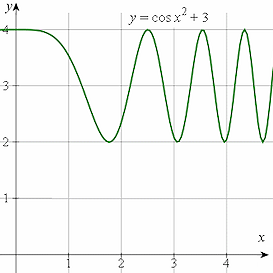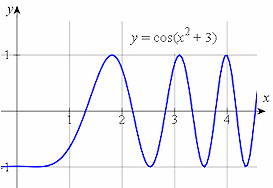Search IntMath
Close

450+ Math Lessons written by Math Professors and Teachers

5 Million+ Students Helped Each Year

1200+ Articles Written by Math Educators and Enthusiasts

Simplifying and Teaching Math for Over 23 Years

# 1. Derivatives of the Sine, Cosine and Tangent Functions

by M. Bourne

It can be shown from first principles that:

(d(sin x))/(dx)=cos x

(d(cos x))/dx=-sin x

(d(tan x))/(dx)=sec^2x

Explore animations of these functions with their derivatives here:

In words, we would say:

The derivative of sin x is cos x,
The derivative of cos x is −sin x (note the negative sign!) and
The derivative of tan x is sec2x.

Now, if u = f(x) is a function of x, then by using the chain rule, we have:

(d(sin u))/(dx)=cos u(du)/(dx)

(d(cos u))/dx=-sin u(du)/(dx)

(d(tan u))/(dx)=sec^2u(du)/(dx)

### Example 1

Differentiate y = sin(x^2 + 3).

First, let: u = x^2+ 3 and so y = sin u.

We have:

(dy)/(dx)=(dy)/(du)(du)/(dx)

=cos u(du)/(dx)

=cos(x^2+3)(d(x^2+3))/(dx)

=2x\ cos(x^2+3)

IMPORTANT:

cos x2 + 3

does not equal

cos(x2 + 3).

The brackets make a big difference. Many students have trouble with this.

Here are the graphs of y = cos x2 + 3 (in green) and y = cos(x2 + 3) (shown in blue).

The first one, y = cos x2 + 3, or y = (cos x2) + 3, means take the curve y = cos x2 and move it up by 3 units.The second one, y = cos(x2 + 3), means find the value (x2 + 3) first, then find the cosine of the result.

They are quite different!### Example 2

Find the derivative of y = cos 3x^4.

Let u = 3x4 and so y = cos u.

Then

(dy)/(dx)=(dy)/(du)(du)/(dx)

=-sin u(du)/(dx)

=-sin(3x^4)(d(3x^4))/(dx)

=-12x^3sin 3x^4

### Example 3

Differentiate y = cos^3 2x

This example has a function of a function of a function.

Let u = 2x and v = cos 2x

So we can write y = v^3 and v = cos\ u

(dy)/(dx)=(dy)/(dv)(dv)/(du)(du)/(dx)

=3v^2(-sin u)(2)

=3(cos^2 2x)(-sin 2x)(2)

=-6\ cos^2 2x\ sin 2x

### Example 4

Find the derivative of y = 3 sin 4x + 5 cos 2x^3.

In the final term, put u = 2x3.

We have:

y=3 sin 4x+5 cos 2x^3

(dy)/(dx)=(3)(cos 4x)(4)+ (5)(-sin 2x^3)(6x^2)

=12 cos 4x-30x^2 sin 2x^3

## Exercises

1. Differentiate y = 4 cos (6x2 + 5).

Put u = 6x2 + 5, so y = 4 cos u.

So

(dy)/(dx)=(dy)/(du)(du)/(dx)

=4[-sin(6x^2+5)][(12x)]

=-48x\ sin(6x^2+5)

2. Find the derivative of y = 3 sin3 (2x4 + 1).

Put u = 2x4 + 1 and v = sin u

So y = 3v3

(dy)/(dx)=(dy)/(dv)(dv)/(du)(du)/(dx)

=[9v^2][cos u][8x^3]

=[9\ sin^2u][cos(2x^4+1)][8x^3]

=72x^3sin^2(2x^4+1)cos(2x^4+1)

3. Differentiate y = (x − cos2x)4.

Put u = x − cos2x and then y = u4.

Now

(du)/(dx)=1-2\ cos x(-sin x)

=1+2\ sin x\ cos x

and

(dy)/(du)=4u^3

So we have:

(dy)/(dx)=(dy)/(du)(du)/(dx)

=4u^3(du)/(dx)

=4[x-cos^2x]^3[1+ {:2 sin x cos x]

4. Find the derivative of:

y=(2x+3)/(sin 4x)

Put u = 2x + 3 and v = sin 4x

Now

(dv)/(dx)=4\ cos 4x

So using the quotient rule, we have:

(dy)/(dx) =(v(du)/(dx)-u(dv)/(dx))/v^2

=((sin 4x)(2)-(2x+3)(4\ cos 4x))/(sin^2 4x)

=(2\ sin 4x-4(2x+3)cos 4x)/(sin^2 4x)

5. Differentiate y = 2x sin x + 2 cos xx2cos x.

First, we write the right hand side as:

y = 2x\ sin x + (2 − x^2) cos x.

We have 2 products. The first term is the product of (2x) and (sin x). The second term is the product of (2-x^2) and (cos x).

So, using the Product Rule on both terms gives us:

(dy)/(dx)= (2x) (cos x) + (sin x)(2) +  [(2 − x^2) (−sin x) + (cos x)(−2x)]

= cos x (2x − 2x) +  (sin x)(2 − 2 + x^2)

= x^2sin x

6. Find the derivative of the implicit function

x cos 2y + sin x cos y = 1.

The implicit function:

x\ cos 2y+sin x\ cos y=1

We differentiate each term from left to right:

x(-2\ sin 2y)((dy)/(dx)) +(cos 2y)(1) +sin x(-sin y(dy)/(dx)) +cos y\ cos x

=0

So

(-2x\ sin 2y-sin x\ sin y)((dy)/(dx)) =-cos 2y-cos y\ cos x

Solving for dy/dx gives us:

(dy)/(dx)=(-cos 2y-cos y\ cos x)/(-2x\ sin 2y-sin x\ sin y)

= (cos 2y+cos x\ cos y)/(2x\ sin 2y+sin x\ sin y)

7. Find the slope of the line tangent to the curve of

y=(2 sin 3x)/x

where x = 0.15

(dy)/(dx)=(x(6\ cos 3x)-(2\ sin 3x)(1))/x^2

=(6x\ cos 3x-2\ sin 3x)/x^2

When x = 0.15 (in radians, of course), this expression (which gives us the slope) equals -2.65.

Here is a graph of our situation. The tangent to the curve at the point where x=0.15 is shown. Its slope is -2.65.8. The current (in amperes) in an amplifier circuit, as a function of the time t (in seconds) is given by

i = 0.10 cos (120πt + π/6).

Find the expression for the voltage across a 2.0 mH inductor in the circuit, given that

V_L=L(di)/(dt)

 V_L =L(di)/(dt)

=0.002(di)/(dt)

=0.002(0.10)(120pi) xx(-sin(120pit+pi/6))

=-0.024pi\ sin(120pit+pi/6)

9. Show that y = cos3x tan x satisfies

cos x(dy)/(dx)+3y sin x-cos^2x=0

The right hand side is a product of (cos x)3 and (tan x).

Now (cos x)3 is a power of a function and so we use Differentiating Powers of a Function:

d/(dx)u^3=3u^2(du)/(dx)

With u = cos x, we have:

d/(dx)(cos x)^3=3(cos x)^2(-sin x)

Now, from our rules above, we have:

d/(dx)tan x=sec^2x

Using the Product Rule and Properties of tan x, we have:

(dy)/(dx)

=[cos^3x\ sec^2x] +tan x[3(cos x)^2(-sin x)]

=(cos^3x)/(cos^2x) +(sin x)/(cos x)[3(cos x)^2(-sin x)]

=cos x-3\ sin^2x\ cos x

We need to determine if this expression creates a true statement when we substitute it into the LHS of the equation given in the question.

 "LHS"

=cos x(dy)/(dx) +3y\ sin x-cos^2x

=cos x(cos x-3\ sin^2x\ cos x) +3(cos^3x\ tan x)sin x-cos^2x

=cos^2x -3\ sin^2x\ cos^2x +3\ sin^2x\ cos^2x -cos^2x

=0

 ="RHS"

We have shown that it is true.

10. Find the derivative of y = x tan x

This is the product of x and tan x.

So we have:

d/(dx)(x\ tan x) =(x)(sec^2x)+(tan x)(1)

=x\ sec^2x+tan x

# Problem Solver

Need help solving a different Trigonometry problem? Try the Problem Solver.

Disclaimer: IntMath.com does not guarantee the accuracy of results. Problem Solver provided by Mathway.

## Problem SolverThis tool combines the power of mathematical computation engine that excels at solving mathematical formulas with the power of GPT large language models to parse and generate natural language. This creates math problem solver thats more accurate than ChatGPT, more flexible than a calculator, and faster answers than a human tutor. Learn More.